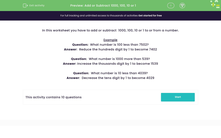# Add or Subtract 1,000, 100, 10 or 1

In this worksheet, students will add or subtract 1,000, 100, 10 or 1 to/from a given number by looking at place value.Key stage:  KS 2

Curriculum topic:   Number: Addition and Subtraction

Curriculum subtopic:   Add/Subtract up to Four-Digit Numbers

Popular topics:   Subtraction worksheets, Addition worksheets, Place Value worksheets

Difficulty level:#### Worksheet Overview

In this activity, we will be adding or subtracting  1,000, 100, 10 or 1 to or from a number.

Example

Question:  What number is 100 less than 7,502?

Answer:  Reduce the hundreds digit by 1 to become 7,402

Question:  What number is 1,000 more than 539?

Answer: Increase the thousands digit by 1 to become 1,539

Question:  What number is 10 less than 4,039?

Answer:  Decrease the tens digit by 1 to become 4,029

Hopefully, you noticed that generally, we only need to change the digit in the appropriate column, the other digits usually stay unchanged.

Shall we have a go at some questions now?### What is EdPlace?

We're your National Curriculum aligned online education content provider helping each child succeed in English, maths and science from year 1 to GCSE. With an EdPlace account you’ll be able to track and measure progress, helping each child achieve their best. We build confidence and attainment by personalising each child’s learning at a level that suits them.

Get started